# Calculate a field

You can add and calculate new fields for your dataset using the data table or data engineering. Calculated fields allow you to create values, such as growth rates, percent loss, and change over time by choosing fields from your dataset and applying operators and functions.

You can also calculate fields using Calculate % Change, Calculate Ratio, and Calculate Z-Score in Find answers.

##### Tip:

Use the data table to add normalized data to your dataset for maps, charts, and tables. After the data is calculated and added to your dataset, change the field type to identify it as a rate/ratio field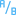. For more information on data normalization, see Choropleth maps.

## Calculate a field in the data table

Fields calculated in the data table only appear in the workbook and are not added to the original dataset. If you want to preserve the calculated field outside of the workbook, you can create a new feature layer by sharing the dataset or using data engineering.

##### Note:

The data table provides a representative view of your data and has a display limit of 2,000 rows. Sorting your table in ascending and descending order allows you to view the top 2,000 rows and the bottom 2,000 rows. All calculations are performed on the full dataset.

Complete the following steps to calculate a field in the data table:

1. Open a workbook and add data, if necessary.
2. From the data pane, click the Dataset options button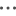next to the dataset where you want to add a calculated field.
3. Click View data table.
4. Click + Field.

A column named New Field is appended to the table.

##### Note:

You can resize and reorder the columns, but these changes are not saved.

5. Click the heading of the new column and provide a more descriptive name.
6. Click fx or Enter calculate function to display a menu with functions, field names, and operators to build your formula.

Equivalent keyboard commands can also be used to replace any of the buttons from the fx menu.

7. Use the functions, fields, and operators to complete your calculation as required.
8. Click Run.

If the Run button is not enabled, there is an error in the syntax of your calculation.

The new calculated field appears at the end of your dataset. You can remove a calculated field by selecting it and clicking the Delete calculated field button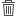.

## Calculate a column using data engineering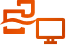##### Note:

Data engineering is available in Insights desktop. All Insights in ArcGIS Online and Insights in ArcGIS Enterprise users have access to Insights desktop. For more information, see Overview of ArcGIS Insights.

Data engineering is currently in Preview.

The Calculate column tool can be added to a data model and used to add columns to the output dataset. Columns calculated in a data workbook will be permanently added to the output dataset, rather than existing only in the workbook.

Complete the following steps to calculate a column using data engineering:

1. Open a data workbook in Insights desktop and add data, if necessary.
2. On the side toolbar, click the Calculate column button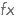.
3. For the New column name parameter, type the name of the new column.
##### Note:

The supported databases in Insights only support letters, numbers, and underscores for column names. Unsupported characters in column names are converted to underscores when saved to a database (for example, population/sq mile is converted to population_sq_mile). For best results, apply a column name that uses only supported characters then update the column alias with other characters, if necessary.

4. In the Column expression box, use the functions, column names, and operators to complete your calculation as required.

Equivalent keyboard commands can also be used to replace any of the buttons.

##### Note:

If the data workbook has more than one dataset, the input options are based on the active table.

5. Click Run.

If the Run button is not enabled, there is an error in the syntax of your calculation.

The new calculated column is added to the table and the Calculate column tool is added to the data model.

## Operators

Calculating a field can require both mathematical and logical operators. The following operators are available for calculating fields:

OperatorUse

+

-

Subtraction.

×

Multiplication. The equivalent keyboard command is *.

÷

Division. The equivalent keyboard command is /.

xy

Power function. The equivalent keyboard command is ^.

<

Less than.

>

Greater than.

=

Equal to.

<=

Less than or equal to.

>=

Greater than or equal to.

<>

Not equal to.

,

Comma, used as a separator between syntax components in functions.

(

Left bracket.

)

Right bracket.

AND

Logical operator where all conditions must be met.

OR

Logical operator where one of the conditions must be met.

IS NULL

A binary operator that tests if values in a field are null. An output value of 0 is assigned when the tested value is not null, and an output value of 1 is assigned when the tested value is null.

IS NOT NULL

A binary operator that tests if values in a field are not null. An output value of 0 is assigned when the tested value is null, and an output value of 1 is assigned when the tested value is not null.

##### Note:

Mathematical operators, such as +, -, >, and =, are compatible with number and rate/ratio fields. Functions, such as CONCATENATE() and DATEDIF(), can be used in place of certain mathematical operators for string fields and date/time fields, respectively.

The IS NULL and IS NOT NULL operators are compatible with all field types (string, number, rate/ratio, and date/time) except locations. Some string fields with null values may be configured as empty strings. In those cases, IS NULL and IS NOT NULL will not return the expected results. Instead, the expressions field="" and field<>"" should be used in place of field IS NULL and field IS NOT NULL, respectively.

The AND and OR operators can be used to join conditions with different syntax than their corresponding logical functions. The following examples show equivalent calculations using the functions and operators:

##### Note:

When calculating fields, AND and OR must be used within the IF() function.

FunctionOperator

IF(AND(MeanAge>=18, MeanAge<=33),"Millennial","Other")

IF(MeanAge>=18 AND MeanAge<=33,"Millennial","Other")

IF(OR(Crime="Theft", Crime="Theft from vehicle", Crime="Shoplifting"), "Larceny", "Other")

IF(Crime="Theft" OR Crime="Theft from vehicle" OR Crime="Shoplifting", "Larceny", "Other")

## Functions

Functions can be accessed using the fx button or the Enter calculate function field in the data table. There are four types of functions: string, numeric, date, and logical.

When you add a function to your calculation, a pop-up displays the function syntax. You can remove the pop-up using the close button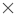, or redisplay the pop-up by clicking the function in the Enter calculate function field.

### String functions

Most string functions use string inputs to produce string outputs. The two exceptions are the VALUE() function and the FIND() function, which both use string inputs to produce numeric outputs.

Input text in string functions can be either literal (text surrounded by quotation marks) or category field values. The following table uses category fields for its examples, along with example values that could be found in those fields. Quotation marks are used in the field values to demonstrate that the fields have categorical data.

SyntaxDescriptionExample

CONCATENATE(text1, [text2], ... )

Concatenates two or more string values.

A dataset of schools in California contains fields for street address, city, and ZIP Code. A single field of mailing addresses can be created using the CONCATENATE() function:

• Function syntax: CONCATENATE(Address,", ", City," , CA, ",ZIP)
• Example field values:
• Address = "380 New York St"
• City = "Redlands"
• ZIP = "92373"
• Result text: "380 New York St, Redlands, CA, 92373"

FIND(find_text, within_text, [start_num])

Gives the position of the specified text (character or characters) within a string or text field. The FIND() function may be most useful when used in conjunction with other functions, such as MID(), LEFT(), or RIGHT().

A dataset contains a field with street addresses (including number and street name). To classify the data by street, the street name has to be removed from the rest of the address using the MID() function. However, each number is a different length, so the start_num will be different for each row. The start_num can be found using the FIND() function:

• Function syntax: FIND(" ", Address)
• Example field values:
• Address = "380 New York St"
• Result number: 4

LEFT(text, [num_chars])

Returns part of a text field.

• num_chars: specifies how many characters of the expression will be returned and should be an integer. The number of characters will be counted from left to right, starting in the first position. If num_chars is not provided, the default will be one character.

A dataset of traffic accidents includes a category field with the day of the accident, including the day of the week, date, and year. To study the accidents by the day of the week, a new field can be calculated to show the first three characters of the field (starting with day of the week) using the LEFT() function:

• Function syntax: LEFT(Accident_Date, 3)
• Example field values:
• Accident_Date = "Monday, November 14, 2016"
• Result text: "Mon"

LOWER(text)

Returns a character expression with all data converted to lowercase.

A public works department is compiling a list of street signs that need to be replaced. As new entries are added to the list, the format of the Status field has become unstandardized, making it difficult to display the signs with unique values. The Status field can be standardized to have all lowercase letters using the LOWER() function:

• Function syntax: LOWER(Status)
• Example field values:
• Status = "Installed"
• Result text: "installed"

MID(text, start_num, [num_chars])

Returns part of a text field.

• start_num: specifies the position of the first character (starting at 1). The start_num must be an integer.
• num_chars: specifies how many characters of the expression will be returned and should be an integer. No empty characters are returned if the num_chars value is greater than the length of the string. If num_chars is not provided, the default will be all the characters after the starting character.

A dataset of schools in California contains fields for street address, city, and ZIP Code. The street name can be isolated from the street address using the MID() function:

• Function syntax: MID(Address, 5, 20)
• Example field values:
• Address = "380 New York St"
• Result text: "New York St"

RIGHT(text, [num_chars])

Returns part of a text field.

• num_chars: specifies how many characters of the expression will be returned and should be an integer. The number of characters will be counted from right to left, starting in the last position. If num_chars is not provided, the default will be one character.

A dataset of national parks includes a field with the name of the park and the two-digit state code. To symbolize the parks by state, a new field can be added and calculated using the RIGHT() function:

• Function syntax: RIGHT(Park, 2)
• Example field values:
• Park = "Hawai'i Volcanoes National Park, HI"
• Result text: "HI"

SUBSTITUTE(source_text, old_text, new_text)

Replaces the old text in a string with the specified new text.

• source_text: The original text or field with text.
• old_text: The text within the source_text that you want to replace.
• new_text: The text to replace the old_text.

A dataset of pothole locations contains a field with street names. The dataset needs to be updated when Main Street is renamed 5th Street. The street_name field can be updated using the SUBSTITUTE() function:

• Function syntax: SUBSTITUTE(street_name, "Main", "5th")

##### Note:

The SUBSTITUTE() function will replace all occurrences of old_text with new_text. For example, in the function SUBSTITUTE("aba", "a", "c") the result text is "cbc".

TRIM(text)

Returns the string with extra spaces removed from the ends.

A feature service contains text fields with extra spaces at the beginning and end of their values. The extra spaces can be removed using the TRIM() function:

• Function syntax: TRIM(City)
• Example field values:
• City = " Redlands "
• Result text: "Redlands"

UPPER(text)

Returns a character expression with all data converted to uppercase.

A dataset with locations of NGO headquarters contains a field with the organizations' full names and their acronyms when applicable. The acronyms can be standardized to have all capital letters using the UPPER() function:

• Function syntax: UPPER(Org)
• Example field values:
• Org = "Nasa"
• Result text: "NASA"

VALUE(text, [format])

Converts text to a number.

• format: specifies the character used as a decimal separator in the number. The format may be either a decimal comma (",") or decimal point ("."). If a format is not provided, the default separator will be a decimal point.

##### Note:

Nested functions within the VALUE() function may output unexpected results if a format is not provided. Therefore, it is recommended that you specify a format when using the VALUE() function.

The VALUE() function does not currently support converting text to negative numbers.

A dataset of retail stores has a category field with the revenue amounts. The Revenue field can be converted to numerical values using the VALUE() function.

• Function syntax: VALUE(Revenue, ".")
• Example field values:
• Revenue = "1,000.00"
• Result number: 1000.00

### Numeric functions

Numeric functions use number inputs to produce number outputs. Number functions are most likely to be used in conjunction with other functions or as a method of transforming data.

Input numbers can be either literal numbers or number fields. Some of the examples below use numbers as input rather than fields to better display the usage of each function.

SyntaxDescriptionExample

ABS(number)

Returns the absolute value.

• Function syntax: ABS(-350)
• Result number: 350

AVG(number)

Returns the mean value.

• Function syntax: AVG(field)
• Example number values for field: 4, 5, 11, 6.5
• Result: 6.63

CEILING(number)

Rounds a number up to the nearest multiple of 1.

• Function syntax: CEILING(7.8)
• Result number: 8

COS(number)

The trigonometric cosine of the specified angle, in radians.

The following formula can be used to convert degrees to radians:

``````radians = θπ/180
where:
θ = the angle in degrees
π ≈ 3.14``````

• Function syntax: COS(0.35)
• Result number: 0.94

FLOOR(number)

Rounds a number down to the nearest multiple of 1.

• Function syntax: FLOOR(7.8)
• Result number: 7

LN(number)

The natural logarithm of the specified float expression. The natural logarithm uses the constant e as the base value (approximately 2.72)

• Function syntax: LN(16)
• Result number: 2.77

LOG(number)

The logarithm of a number to a specific base. The default is base 10.

• Function syntax: LOG(16, 2)
• Result number: 4

MAX(number) or MAX(date)

Returns the maximum value.

• Function syntax: MAX(num_field)
• Example values for num_field: 4, 5, 11, 6.5
• Result: 11

MIN(number) or MIN(date)

Returns the minimum value.

• Function syntax: MIN(date_field)
• Example values for date_field: 03/17/2018, 02/14/2019, 10/31/2020
• Result: 03/17/2018

POWER(number, power)

The value of the expression raised to the specified power.

• Function syntax: POWER(2, 4)
• Result number: 16

ROUND(number, num_digits)

Rounds numeric values to the specified number of digits.

• num_digits = the number of decimal places in the output
• If num_digits is positive, the number is rounded to the corresponding number of decimal places
• If num_digits is zero, the number is rounded to the nearest integer
• If num_digits is negative, the number is rounded to the specified number of digits left of the decimal point

• Function syntax: ROUND(54.854827, 2)
• Result number: 54.85
• Function syntax: ROUND(54.854827, -1)
• Result number: 50

SIN(number)

The trigonometric sine of the specified angle, in radians.

The following formula can be used to convert degrees to radians:

``````radians = θπ/180
where:
θ = the angle in degrees
π ≈ 3.14``````

• Function syntax: SIN(0.79)
• Result number: 0.71

STDEV(number)

The standard deviation of a number field.

• Function syntax: STDEV(field)
• Example number values for field: 4, 5, 11, 6.5
• Result: 3.09

SUM(number)

Returns the total value.

• Function syntax: SUM(field)
• Example number values for field: 4, 5, 11, 6.5
• Result: 26.5

TAN(number)

Returns the tangent of the input expression.

The following formula can be used to convert degrees to radians:

``````radians = θπ/180
where:
θ = the angle in degrees
π ≈ 3.14``````

• Function syntax: TAN(1.05)
• Result number: 1.74

### Date functions

Date functions can use date fields, text, or no input, depending on which function is being used. The DATEVALUE() function can be used to replace a date field in the DATEDIF() or TIMEDIF() function.

##### Note:

Date/time fields used in DATEDIF() and DATEADD() must be in a format that includes a date (in other words, either date and time or date only). Time-only date/time fields will not be accepted.

Date/time fields used in TIMEDIF() must be in a format that includes a time (in other words, either date and time or time only). Date-only date/time fields will not be accepted.

SyntaxDescriptionExample

DATE(year, month, day, [hour, minute, second], [AM/PM])

Creates a date/time field using three or more separate fields or values. The following specifications are accepted:

• year: "YY" (two-digit year) or "YYYY" (four-digit year)
• month: "MM" (1–12)
• day: "DD" (1–31)
• hour: "HH" (0–23) or "hh" (1–12)
• minute: "mm" (0–59)
• second: "ss" (0–59)
• AM/PM: "AM"/"PM" (not case sensitive)

##### Note:

All input fields can be numbers or strings, except the AM/PM parameter. Numbers must be integer values. Two-digit years must be strings.

If you are using the DATE() function with a database dataset and you have specified any time parameters (hour, minute, or second), you must enter an input value for all time parameters.

A dataset of restaurants includes string fields with the date and time of the most recent health inspections. A date field for the inspections can be created using the DATE() function:

• Function syntax: DATE(2016, Month, Day, Hour, Min, 00)
• Example field values:
• Month = 8
• Day = 15
• Hour = 11
• Minute = 30
• Result date: 8/15/2016, 11:30:00

##### Note:

The result dates in this example will be formatted using a 24-hour clock. To use a 12-hour clock, you must specify AM or PM. The same example function can be written as DATE(2016, Month, Day, Hour, Min, 00, "AM").

TIME(hour, minute, second)

Creates a date/time field using three separate fields or values. The following specifications are accepted:

• hour: "HH" (0–23) or "hh" (1–12)
• minute: "mm" (0–59)
• second: "ss" (0–59)
• AM/PM: "AM"/"PM" (not case sensitive)

##### Note:

All input fields can be numbers or strings, except the AM/PM parameter. Numbers must be integer values.

A dataset of hospital records includes patient arrival times. A time field for the records can be created using the TIME() function:

• Function syntax: TIME(Hour, Min, 00)
• Example field values:
• Hour = 11
• Minute = 30
• Result time: 11:30:00

##### Note:

The result times in this example will be formatted using a 24-hour clock. To use a 12-hour clock, you must specify AM or PM. The same example function can be written as TIME(Hour, Min, 00, "AM").

Adds a specified amount of time to the dates in the input field.

• date must be a date/time field. The field must be in a format that includes a date (in other words, either date and time or date only). Time-only date/time fields will not be accepted.
• integer: The number to add to the input date. If a constant is used, the number must be an integer and can be positive or negative. If a number field is used, decimal places will be ignored. Therefore, it is best practice to use a field that contains only integers or calculate a new field with integers (for example, by using the ROUND() function).
• unit: The unit of time to be added. The supported unit values include the following:
• "ss" = seconds
• "mm" = minutes
• "h" = hours
• "D" = days (default)
• "M" = months
• "Y" = years

If no unit is provided, "D" will be used.

When months are added to a date/time field, the output will be the same day of the month, regardless of the number of days in the month. If the corresponding day of the month is not valid in the new month, the last day of the month is used. For example, if a calculation adds three months to the input field, and one of the original dates is November 30, 2021, the output value of February 30, 2022 is not valid. The result will be recorded as February 28, 2022, since 2022 is not a leap year.

A public health unit is responsible for keeping vaccine records for patients within the health district. A specific vaccine has two doses that must be taken at least 60 days apart and no more than 180 days apart. DATEADD() can be used to create fields with the first date the patients are eligible for their second dose and the deadline for the final dose.

Eligibility date:

• Function syntax: DATEADD(first_dose_date, 60, "D")
• Example field values:
• first_dose_date = 7/11/2021
• Result date: 9/9/2021

Final date:

• Function syntax: DATEADD(first_dose_date, 180, "D")
• Example field values:
• first_dose_date = 7/11/2021
• Result date: 1/7/2022

Adds a specified amount of time to the times in the input field.

• time must be a date/time field. Time can be added to all date/time fields, including fields that do not already include a time component.
• integer: The number to add to the input time. If a constant is used, the number must be an integer and can be positive or negative. If a number field is used, decimal places will be ignored. Therefore, it is best practice to use a field that contains only integers or calculate a new field with integers (for example, by using the ROUND() function).
• unit: The unit of time to be added. The supported unit values include the following:
• "ss" = seconds
• "mm" = minutes
• "h" = hours

A dataset has dates and times recorded in coordinated universal time (UTC) and needs to be converted to central standard time (CST). A date/time field with times in CST can be created using the TIMEADD() function:

• Function syntax: TIMEADD(UTC_date, -6, "h")
• Example field values:
• UTC_date = 7/11/2021 2:30:00 PM
• Result date: 7/11/2021 8:30:00 AM

DATEDIF(start_date, end_date, [unit])

Calculates the elapsed time between two dates. The start_date must take place before the end_date; otherwise, the output will be a negative value.

• start_date and end_date can be date/time fields or a DATEVALUE() function. If date/time fields are used, the field must be in a format that includes a date (in other words, either date and time or date only). Time-only date/time fields will not be accepted.
• unit: The unit of time to be returned. The supported unit values include the following:
• "ss" = seconds
• "mm" = minutes
• "h" = hours
• "D" = days (default)
• "M" = months
• "Y" = years

If no unit is provided, "D" will be used.

Rounding is performed on the results for each unit as follows:

• Seconds—Rounding is not performed for seconds.
• Minutes—One minute is counted when a full 60 seconds takes place between dates. Results will be rounded down for partial minutes.
• Hours—One hour is counted when a full 60 minutes takes place between dates. Results will be rounded down for partial hours.
• Days—One day is counted when a full 24 hours takes place between dates. Results will be rounded down for partial days.
• Months—One month is counted when a full month takes place between dates (for example, January 1 to February 1), regardless of how many days are in the specific months. Results will be rounded down for partial months. Time components, such as hours, are not considered in the calculation.
• Year—One year is counted when 183 days (half a year) take place between dates. Results will be rounded down for partial years consisting of fewer than 183 full days.

A dataset of restaurants includes the dates of their most recent health inspections. The number of days elapsed between health inspections can be calculated using the DATEDIF() function:

Example 1:

• Function syntax: DATEDIF(Inspection1, Inspection2, "D")
• Example field values:
• Inspection1 = 8/15/2016, 11:30:00 AM
• Inspection2 = 10/31/2016 2:30:00 PM
• Result time (days): 77

Example 2:

• Function syntax: DATEDIF(Inspection1, Inspection2, "D")
• Example field values:
• Inspection1 = 8/15/2016, 11:30:00 AM
• Inspection2 = 8/16/2016 8:00:00 AM
• Result time (days): 0

Example 3:

• Function syntax: DATEDIF(Inspection1, Inspection2, "M")
• Example field values:
• Inspection1 = 8/15/2016, 11:30:00 AM
• Inspection2 = 10/31/2016 2:30:00 PM
• Result time (months): 2

TIMEDIF(start_time, end_time, [unit])

Calculates the elapsed time between two date/time values. The start_time must take place before the end_time; otherwise, the output will be a negative value.

• start_time and end_time can be date/time fields or a DATEVALUE() function. If a time is used (for example, hh:mm:ss), both the start_time and end_time must be in a time-only format.
• unit: The unit of time to be returned. The supported unit values include the following:
• "ss" = seconds (default)
• "mm" = minutes
• "h" = hours

If no unit is provided, "ss" will be used.

Rounding is performed on the results for each unit as follows:

• Seconds—Rounding is not performed for seconds.
• Minutes—One minute is counted when a full 60 seconds takes place between dates. Results will be rounded down for partial minutes.
• Hours—One hour is counted when a full 60 minutes takes place between dates. Results will be rounded down for partial hours.

A dataset of hospital operation records includes the start and end time of surgeries. The time each patient spends in surgery can be calculated using the TIMEDIF() function:

• Function syntax: TIMEDIF(TimeIn, TimeOut, "mm")
• Example field values:
• TimeIn = 11:30:00 AM
• TimeOut = 2:30:20 PM
• Result time (minutes): 180

DATEVALUE(date_text, [format])

Converts text to a date.

• date_text can be a string field or text entered inside quotation marks.
• format (optional): The format of the date entered. The format is entered as text surrounded by quotation marks. The format can be entered using the following unit specifications:
• "MM" - Month (1–12)
• "DD" - Day of the month (1–31)
• "YY" - Two-digit year
• "YYYY" - Four-digit year
• "HH" - Hour (0–23)
• "hh" - Hour (1–12)
• "mm" - Minutes (0–59)
• "ss" - Seconds (0–59)
• "AM"/"PM" - Not case sensitive

##### Note:

Nested functions within the DATEVALUE() function may output unexpected results if a format is not provided. Therefore, it is recommended that you specify a format when nesting functions within DATEVALUE().

Dates specified without separators (for example, "10312016") will be treated as milliseconds if no format is provided. Therefore, it is recommended that you specify a format when entering dates without separators.

For dates specified with separators but without a format, the format will be guessed. If the format cannot be guessed, the default "MM-DD-YY" will be applied.

The DATEVALUE() function can only read text entered inside quotation marks or string fields. A date/time field cannot be used as input to the DATEVALUE() function.

A dataset of restaurants includes the dates of their most recent health inspections. The number of days elapsed since the last inspection can be calculated using the DATEVALUE() function to specify the current date:

• Function syntax: DATEDIF(Inspection_date, DATEVALUE("10/31/2016", "MM/DD/YYYY"), "D")
• Example field values:
• Inspection_date = 8/15/2016, 11:30:00 AM
• Result number: 77

Dates and times can be formatted in several ways. The following examples are some of the ways you can format text into dates:

• DATEVALUE("12/25/2016 12:30:25 pm", "MM/DD/YYYY hh:mm:ss pm")
• DATEVALUE("12/25/2016 14:23:45", "MM/DD/YYYY HH:mm:ss")
• DATEVALUE("25-08-2008 08:40:13 AM", "DD/MM/YYYY hh:mm:ss AM")

NOW()

Returns the current date and time in date/time format. The time is recorded based on coordinated universal time (UTC).

A dataset of restaurants includes the dates of their most recent health inspections. The number of days elapsed since the last inspection can be calculated using the NOW() function to specify the current date:

• Function syntax: DATEDIF(Inspection_date, NOW(), "D")
• Example field values:
• Inspection_date= 8/15/2016, 11:30:00 AM
• Result number: 77

### Logical functions

Logical functions use string or number inputs to produce string or number outputs. The inputs can be either literal (text surrounded by quotation marks or constant numbers) or field values. The following table uses a combination of field and literal values for its examples, along with example values that could be found in the given fields.

SyntaxDescriptionExample

AND(condition,condition, ...)

Tests two or more conditions and returns TRUE if all conditions are met. AND() should be used within the condition parameter of the IF() function.

• condition: Any expression that includes at least one conditional operator. The condition can include numeric, string, and field values. The following conditional operators can be used:
• >: greater than
• <: less than
• >=: greater than or equal to
• <=: less than or equal to
• =: equal to
• <>: not equal to

A dataset contains the mean age of people living within each block group. A market researcher wants to know which block groups contain mostly millennials. The block groups with a mean age within the age range for millennials can be found using a combination of the IF() and AND() functions:

• Function syntax: IF(AND(MeanAge>=18, MeanAge<=33),"Millennial","Other")
• Example field value:
• MeanAge = 43
• Result text: "Other"

IF(condition, TRUE_expression, [FALSE_expression])

Tests a condition and returns a TRUE or FALSE value based on the result.

• condition: Any expression that includes at least one conditional operator. The condition can include numeric, string, and field values. The following conditional operators can be used:
• >: greater than
• <: less than
• >=: greater than or equal to
• <=: less than or equal to
• =: equal to
• <>: not equal to
• TRUE_expression: The expression that will be used if the condition is true. This parameter is mandatory.
• FALSE_expression: The expression that will be used if the condition is false. If no FALSE_expression is provided, the default will be null.

TRUE_expression and FALSE_expression can be any valid expression, including a nested IF() function. The data type for TRUE_expression and FALSE_expression should be the same type (for example, both strings or both numbers).

A dataset contains fields with store revenue and expenses, which can be used to find the status of the stores (whether they have posted a net profit or a net loss). The status of each store can be found using the IF() function:

• Function syntax: IF(Revenue>Expenses, "Profit","Loss")
• Example field values:
• Revenue = 400,000
• Expenses = 350,000
• Result text: "Profit"

ISNULL(field)*

Tests if values in a field are null. An output value of 0 is assigned when the tested value is not null, and an output value of 1 is assigned when the tested value is null.

• field: Supports string, number, rate/ratio, and date/time field types.

ISNULL() can be used on its own, or within the condition parameter of the IF() function.

A dataset contains locations of traffic collisions involving cyclists, as well as the type of bike route present on the street where the collision took place. For collisions that took place on a street without a bike route, the RouteType field is left blank. The dataset can be updated to fill in null values using a combination of the IF() and ISNULL() functions:

• Function syntax: IF(ISNULL(RouteType), "None", RouteType)

ISNOTNULL(field)*

Tests if values in a field are not null. An output value of 0 is assigned when the tested value is null, and an output value of 1 is assigned when the tested value is not null.

• field: supports string, number, rate/ratio, and date/time field types.

ISNOTNULL() can be used on its own, or within the condition parameter of the IF() function.

A dataset contains locations of traffic collisions involving cyclists, as well as the type of bike route present on the street where the collision took place. For collisions that took place on a street without a bike route, the RouteType field is left blank. The dataset can be updated to fill in null values using a combination of the IF() and ISNOTNULL() functions:

• Function syntax: IF(ISNOTNULL(RouteType), RouteType, "None")

OR(condition,condition, ...)

Tests two or more conditions and returns TRUE if at least one condition is met. OR() should be used within the condition parameter of the IF() function.

• condition: Any expression that includes at least one conditional operator. The condition can include numeric, string, and field values. The following conditional operators can be used:
• >: greater than
• <: less than
• >=: greater than or equal to
• <=: less than or equal to
• =: equal to
• <>: not equal to

A dataset contains locations of crimes across a city. A crime analyst wants to analyze the pattern of larceny compared to other similar crimes. To compare larceny to other crimes, the analyst must first determine whether or not each crime was considered larceny. The type of crime can be determined using a combination of the IF() and OR() functions:

• Function syntax: IF(OR(Crime="Theft", Crime="Theft from vehicle", Crime="Shoplifting"), "Larceny", "Other")
• Result text: "Larceny"

*Some fields with no data may be configured as empty rather than null. In those cases, ISNULL() and ISNOTNULL() will not return the expected results. Instead, the expressions field="" and field<>"" should be used in place of ISNULL(field) and ISNOTNULL(field), respectively.